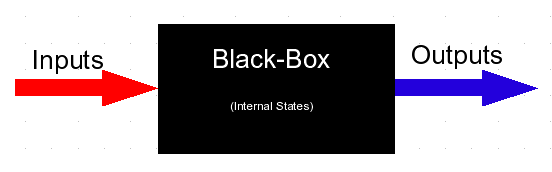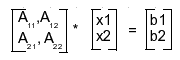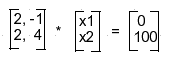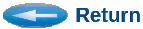# LS Equation Solver, Curve-Fitter

Often a complicated system or component, or some aspects of it, can be modeled by an equation, or set of equations. The equations relate the values of input stimuli, and internal state values, to the component's output values and changes in internal state values.Sometimes this method is called the Black-Box modeling method, or modeling a Black-Box, because it attempts to fit a functional or behaviorally descriptive equation, without actually knowing or describing all the internal details or structures within the Black-Box.

In many actual situations, the internal details of a system or component cannot be known, but measurements can be observed of its response to various stimuli. The least-squares equation solver (LS-Solver) method enables developing model-based representations of such systems.

If the system (or component) is a linear system, its response can be predicted as an additive combination of the input or state terms, each multiplied by a coefficient, such as:
f(x) = 3 * x + 7 * x2

To characterize a black-box system, a series of measurements are taken for various combinations of input stimulus. Both the input and output values are recorded. Alternatively, to design a system with specific behavior, the desired outputs to given input values can be listed. In either case, to determine the coefficients uniquely describing the system, the number of measurement observations, M, must be equal to, or greater than, the number of input stimuli, N.

For systems containing measurement error, or noise, or in cases where the input values are not always independently related, it is often helpful to collect many more than N observations (M >> N).

The LS-Solver accepts the input values and output measurements, and it solves for a set of coefficients that produce estimated outputs that best match the observations. This is also called curve fitting. The match is best in the least-squares sense. That is, the sum of the squares of the differences (errors) between the estimated values and observed values, is minimized.

If the system is exactly determined by the solved coefficients, the coefficients are said to be an exact solution.

The least-square solution method is also useful for designing new systems to match a desired output curve. It can determine the coefficients needed to meet required operating criteria. So it is useful not just for characterizing existing systems.

It can also be used to simplify, or to abstract, a more complex system or model.

There are many reasons the estimated system may not match the actual or intended system over all ranges. For example, the actual system may have-, or the intended system may need-, more terms than you supplied. Or it may have-, or require-, so called hidden internal states. Therefore, this method is also a way to find hidden states, or to determine which inputs the output really dependents on.

The LS-Solver is implemented using the classic least-squares solution method. It is known to be the fastest and most accurate method to solve linear systems. However, it is also known to become unstable under certain circumstances. Therefore, a technique known as diagonal-loading can be used to increase stability for ill-conditioned systems.

If you supply output values that are not truly independent of each other (IE. they are merely a simple multiple of another), then the system is said to be rank-deficient. In other words, you do not need so many coefficients. You will receive an error message from the solution algorithm. Other times this can be caused by measurement errors in the input or output values, which causes the data to produce an ill-conditioned matrix. Fortunately these situations are fairly rare in practice. However, there are number of things you can do to resolve these, should they occur.

The default LS-Solver contains a small amount of diagonal loading. If you do not see a sufficiently close fit (low total error sum), then you may try decreasing the loading. For exactly determined systems, the loading prevents an exact solution. If your system is under-rank or ill-conditioned, you should increase the loading.

The least-squares solution also assumes that your system can be characterized by a smooth, continuously differentiable, performance surface. Many real and analog systems belong to this class. However, many other systems are outside that characterization, or only match within distinct sub-regions. Some discrete or digital systems have outputs that are flat for large ranges, and then instantly switch to new planes at other ranges. Such systems are said to be not continuously differentiable, and are generally not appropriately solved with least-squares methods. However, least-squares can still be applied with the understanding that you will receive the best approximation than can be achieved as a linear sum. It will generally have the greatest error near the transients, but may develop an overall satisfactory approximation, or average simplification, for some applications. Piecewise solutions may also be employed in these situations, where separate solutions are determined for each region.

More extreme situations exist where the output space, or performance surface, is completely non-continuous and non-differentiable. Each output value varies discretely from its neighboring values. Such surfaces are sometimes said to resemble blades of grass on a lawn (that needs cutting). Again, the LS-solver is not intended for such systems, but will produce a continuous approximation. Sometimes such models are acceptable because it may produce an approximation that is statistically accurate.

Similar solvers are contained in other products, such as Matlab, or can be constructed with LAPACK routines. However, the LS-Solver is provided here as a convenience, self-contained utility within the common package. It is helpful especially when other methods are not available, and can be embedded easily within other products.

```

```

## Usage:

The LS-Solver uses the Num-Utils library. It assumes each observation or experiment, j, is expressed as equations of the form:
A[j,1] * x1 + A[j,2] * x2 = b[j]
The equations are assembled into the rows of a matrix, A[,] and vector b[]. For example, two equations would produce the following rows:
A[1,1] * x1 + A[1,2] * x2 = b
A[2,1] * x1 + A[2,2] * x2 = b

To use the LS-Solver, construct a matrix consisting of the A[,] terms into a file called:     A.dat
And vector file of the b[] terms:     b.dat

Then solve for the vector x (which will contain x1 and x2), with the solver script:

In POSIX (Linux/Unix):
source \$CSIM_MODEL_LIBS/solver/solver.com

Or, in Microsoft:
%CSIM_MODEL_LIBS%\solver\solver.bat

The results are placed in file:     X.dat

The solver script consists of the following Num-Utils commands:

```	transpose  A.dat  A.transpose
matmul     A.transpose  A.dat  A.new
identity_matrix -sizeof A.new  -scale 1e-3  ident.dat
matinvert  A1.dat  lterm.inv
matmul     A.transpose  b.dat  rterm.dat
matmul     lterm.inv  rterm.dat  X.dat
more       X.dat
```
If your matrix is not well conditioned or not full-rank, it is recommended that you vary the amount of diagonal loading by changing the scale value in the third command. Basically, you should raise it until you get a result without error messages. You should lower it to minimize estimation error, but not so low that you get error messages. If your measurements are exact or theoretical and your system is full-rank and well-conditioned, you can try setting the scale to zero.
```

```

## How to Construct A.dat and b.dat Files:

Each Num-Utils data file is a simple text-file containing a header-line followed by lines of numbers that represent the vector or matrix data contents.

There are several ways to construct the input files.

1. entervec - This Num-Utils program prompts you to enter a vector or matrix interactively.

2. nums2vec - This program converts a simple list of numbers, such as from Matlab or Excel to NumUtils Real Vector format, by adding the header line and an index column. The matrix can be built up by successive calls to augmatvec.

3. Or, use a text editor to add the header line to CSV or tab-separated-values files, as follows.
```	A.dat header:
!<Type> Real Matrix[2,2] </Type>

!<Type> Real Vector </Type>

Example:
A.dat:
!<Type> Real Matrix[2,2] </Type>
1 1     2
1 2     -1
2 1     2
2 2     4

b.dat:
!<Type> Real Vector </Type>
0 0
1 100

The above data would correspond to the matrix-vector equation:Which would be:And would correspond to the equations:
2 * x1 + (-1) * x2 = 0
2 * x1 +   4  * x2 = 100

After running the LS-Solver script, to solve for the unknown x-values, on these files, it produces:
X.dat:
!<Type> Real Vector </Type>
0 10.0
1 20.0

In other words, the value of x1 = 10.0, and x2 = 20.0, solves the two equations above.

```
```

```

## Future Plans:

This LS-Solver is the first step in our plans to construct a comprehensive fully automatic solver tool. In the future, we will be adding additional solver types that will support the characterization of a wider set of system types, such as non-differentiable, or non-linear dynamic systems. We will also be providing an automatic manager that can characterize your system, and apply the best combination of solvers and coefficient dimensions.